京山游侠

posts - 62, comments - 398, trackbacks - 2, articles - 0
博客园 :: 首页 :: 新随笔 :: 联系 :: 订阅:: 管理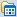先贴代码和结论

#include <stdio.h>
#include <stdlib.h>
#include <memory.h>
#include <sys/time.h>
#include <cuda_runtime.h>
#include <cublas_v2.h>

#define M 2000
#define N 2000
#define K 2000

#define idx(i, j, m) ((j) * (m) + (i))

typedef struct _matrix {
size_t height;
size_t width;
float *elements;
} Matrix;

Matrix empty(size_t m, size_t n){
Matrix temp = {m, n, NULL};
temp.elements = (float*)malloc( m*n*sizeof(float) );
return temp;
}

Matrix zeros(size_t m, size_t n){
Matrix temp = empty(m, n);
memset(temp.elements, 0, sizeof( m*n*sizeof(float) ));
return temp;
}

Matrix rands(size_t m, size_t n){
Matrix temp = empty(m, n);
for(size_t i=0; i<m*n; i++){
temp.elements[i] = (float)rand()/RAND_MAX;
}
return temp;
}

void deleteMatrix(Matrix *mat){
mat->height = 0;
mat->width = 0;
free(mat->elements);
mat->elements = NULL;
}

void printRow(Matrix mat, size_t i){
size_t m = mat.height;
size_t n = mat.width;
if(n < 7){
for(size_t j=0; j<n; j++){
printf(" %7.4f ", mat.elements[idx(i, j, m)]);
}
}else{
for(size_t j=0; j<3; j++){
printf(" %7.4f ", mat.elements[idx(i, j, m)]);
}
printf("   ...   ");
for(size_t j=n-3; j<n; j++){
printf(" %7.4f ", mat.elements[idx(i, j, m)]);
}
}
printf("\n");
}

void printMatrix(Matrix mat){
size_t m = mat.height;
if(m < 7){
for(size_t i=0; i<m; i++){
printRow(mat, i);
}
}else{
for(size_t i=0; i<3; i++){
printRow(mat, i);
}
printf("   ...   \n");
for(size_t i=m-3; i<m; i++){
printRow(mat, i);
}
}
printf("\n");
}

Matrix matMul(Matrix A, Matrix B){
size_t m, n, k;
Matrix C = {0, 0, NULL};
if(A.width == B.height){
m = A.height;
n = B.width;
k = B.height;
C = zeros(m, n);
for(size_t i=0; i<m; i++){
for(size_t j=0; j<n; j++){
float sum = 0;
for(size_t p=0; p<k; p++){
sum += A.elements[idx(i, p, m)] * B.elements[idx(p, j, k)];
}
C.elements[idx(i, j, m)] = sum;
}
}
}else{
printf("Matrix shape error!\n");
}
return C;
}

long getTimer(struct timeval start, struct timeval end){
return (end.tv_sec - start.tv_sec)*1000000 + (end.tv_usec - start.tv_usec);
}

void printTimer(long timer){
printf("%ld,%ld,%ld us\n", timer/1000000, (timer/1000)%1000, timer%1000);
}

bool initCUBLAS(cublasHandle_t *handle){
int count;
cudaGetDeviceCount(&count);
if(count == 0){
printf("There is no device.\n");
return false;
}else{
printf("There is %d device.\n", count);
}
int i;
for(i=0; i<count; i++){
if(cudaGetDeviceProperties(&prop, i) == cudaSuccess){
if(prop.major >= 1){
break;
}
}
}

if(i == count){
printf("There is no device supporting CUDA 1.x.\n");
return false;
}
cudaSetDevice(i);

cublasStatus_t stat;
stat = cublasCreate(handle);
if(stat!=CUBLAS_STATUS_SUCCESS){
printf("CUBLAS initialization failed\n");
return false;
}
printf("CUBLAS initialized.\n");

return true;
}

__global__ void cudaMatMul(Matrix devA, Matrix devB, Matrix devC){
size_t m = devA.height;
size_t k = devB.height;
size_t n = devB.width;

size_t j = blockIdx.y * blockDim.y + threadIdx.y;
size_t i = blockIdx.x * blockDim.x + threadIdx.x;

if(i<m && j<n){
float sum = 0.0;
for(size_t p=0; p<k; p++){
sum += devA.elements[idx(i, p, m)] * devB.elements[idx(p, j, k)];
}
devC.elements[idx(i, j, m)] = sum;
}
}

int main(int argc, char** argv){
struct timeval start;
struct timeval end;

//第一步，创建两个随机矩阵，使用 CPU 计算它们相乘，并计时
gettimeofday(&start, NULL);
Matrix A = rands(M, K);
printf("Matrix A, shape: %ldx%ld, address in memory:%ld\n", A.height, A.width, (size_t)A.elements);
printMatrix(A);

Matrix B = rands(K, N);
printf("Matrix B, shape: %ldx%ld, address in memory:%ld\n", B.height, B.width, (size_t)B.elements);
printMatrix(B);

Matrix C = matMul(A, B);
printf("Matrix C, shape: %ldx%ld, address in memory:%ld\n", C.height, C.width, (size_t)C.elements);
printMatrix(C);
gettimeofday(&end, NULL);
printf("CPU 计算矩阵相乘，用时:");
long timer1 = getTimer(start, end);
printTimer(timer1);

//第二步，将矩阵 A 和 B 拷贝到显卡中，利用显卡计算矩阵乘法，再将结果考回 C 中，并计时
cublasHandle_t handle;
if(!initCUBLAS(&handle)){
return EXIT_FAILURE;
}
gettimeofday(&start, NULL);
size_t m = A.height;
size_t n = B.width;
size_t k = B.height;
Matrix devA = {m, k, NULL};
Matrix devB = {k, n, NULL};
Matrix devC = {m, n, NULL};
cudaMalloc(&devA.elements, m*k*sizeof(float));
cudaMemcpy(devA.elements, A.elements, m*k*sizeof(float), cudaMemcpyHostToDevice);
cudaMalloc(&devB.elements, k*n*sizeof(float));
cudaMemcpy(devB.elements, B.elements, k*n*sizeof(float), cudaMemcpyHostToDevice);
cudaMalloc(&devC.elements, m*n*sizeof(float));
cudaMemset(devC.elements, 0, m*n*sizeof(float));
//调用 GPU 进行计算
size_t blockSize = 32;
size_t gridWidth = (n + blockSize -1)/blockSize;
size_t gridHeight = (m + blockSize -1)/blockSize;
cudaMatMul<<<dim3(gridHeight, gridWidth), dim3(blockSize, blockSize)>>>(devA, devB, devC);
//从 GPU 取回数据
deleteMatrix(&C);
C = zeros(m, n);
printf("从显卡取回数据之前的矩阵 C\n");
printf("Matrix C, shape: %ldx%ld, address in memory:%ld\n", C.height, C.width, (size_t)C.elements);
printMatrix(C);
cudaMemcpy(C.elements, devC.elements, m*n*sizeof(float), cudaMemcpyDeviceToHost);
printf("从显卡取回数据之后的矩阵 C\n");
printf("Matrix C, shape: %ldx%ld, address in memory:%ld\n", C.height, C.width, (size_t)C.elements);
printMatrix(C);

gettimeofday(&end, NULL);
printf("自己写 CUDA 代码，利用 GPU 计算矩阵相乘，用时:");
long timer2 = getTimer(start, end);
printTimer(timer2);

//第三步，直接利用 cublas 库计算矩阵乘法，并计时
gettimeofday(&start, NULL);
float scalar = 1.0;
Matrix devA2 = {m, k, NULL};
Matrix devB2 = {k, n, NULL};
Matrix devC2 = {m, n, NULL};
cudaMalloc(&devA2.elements, m*k*sizeof(float));
cudaMalloc(&devB2.elements, k*n*sizeof(float));
cudaMalloc(&devC2.elements, m*n*sizeof(float));

Matrix C2 = zeros(m, n);

cublasSetMatrix(m, k, sizeof(float), A.elements, m, devA2.elements, m);
cublasSetMatrix(k, n, sizeof(float), B.elements, k, devB2.elements, k);
cublasSetMatrix(m, n, sizeof(float), C2.elements, m, devC2.elements, m);

cublasSgemm(handle, CUBLAS_OP_N, CUBLAS_OP_N, m, n, k, &scalar, devA2.elements, m, devB2.elements, k, &scalar, devC2.elements, m);

printf("从显卡取回数据之前的矩阵 C2\n");
printf("Matrix C2, shape: %ldx%ld, address in memory:%ld\n", C2.height, C2.width, (size_t)C2.elements);
printMatrix(C2);

cublasGetMatrix(m, n, sizeof(float), devC2.elements, m, C2.elements, m);

printf("从显卡取回数据之后的矩阵 C2\n");
printf("Matrix C2, shape: %ldx%ld, address in memory:%ld\n", C2.height, C2.width, (size_t)C2.elements);
printMatrix(C2);
gettimeofday(&end, NULL);
long timer3 = getTimer(start, end);
printf("直接使用 Nvidia 提供的 cuBlas 库进行矩阵乘法计算，用时：");
printTimer(timer3);

printf("\n");
printf("自己写 CUDA 代码利用 GPU 计算矩阵乘法，速度是 CPU 的 %f 倍，利用 cuBlass 库进行计算，速度是 CPU 的 %f 倍。\n",
(float)timer1/timer2, (float)timer1/timer3);
return 0;
}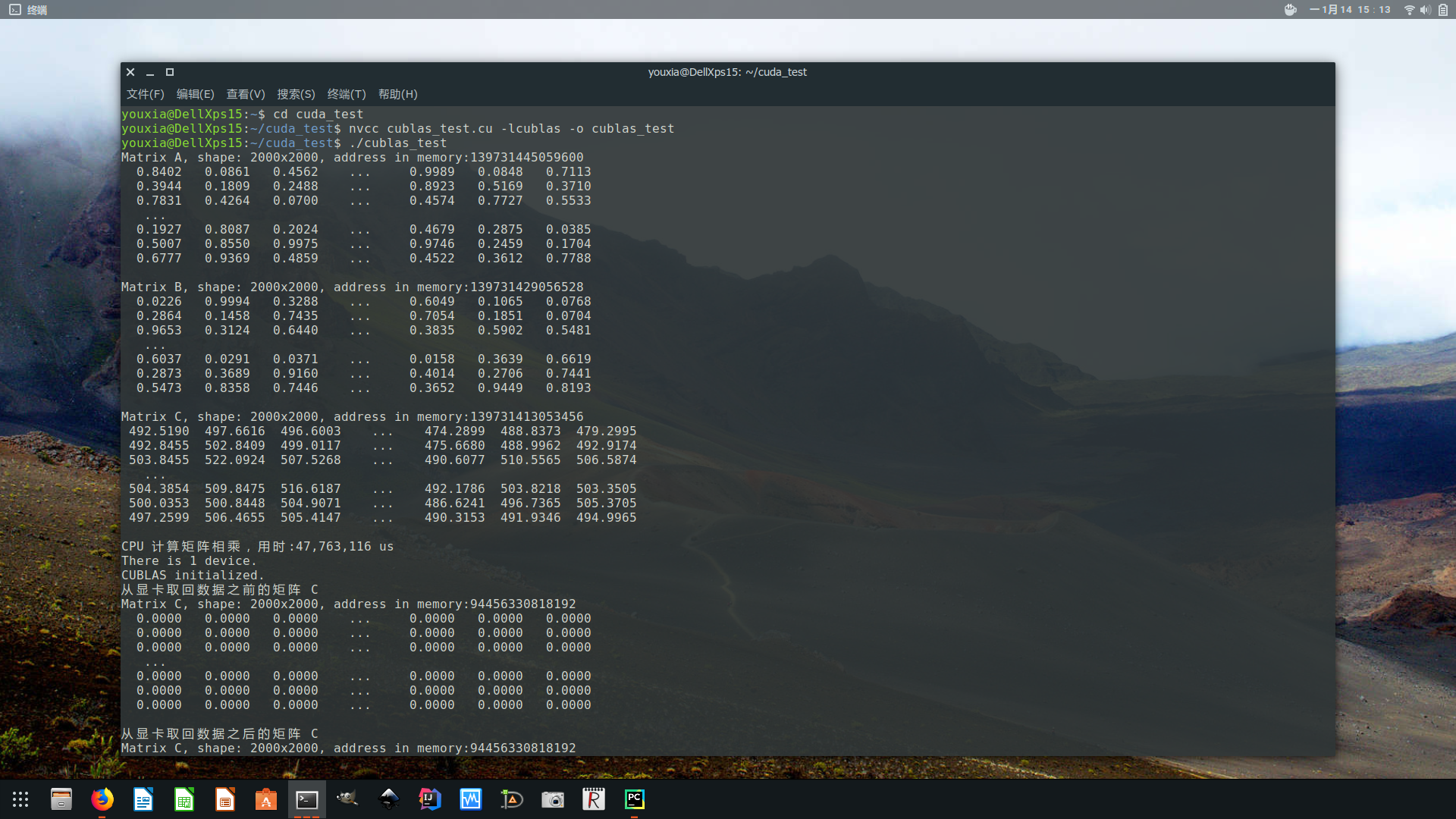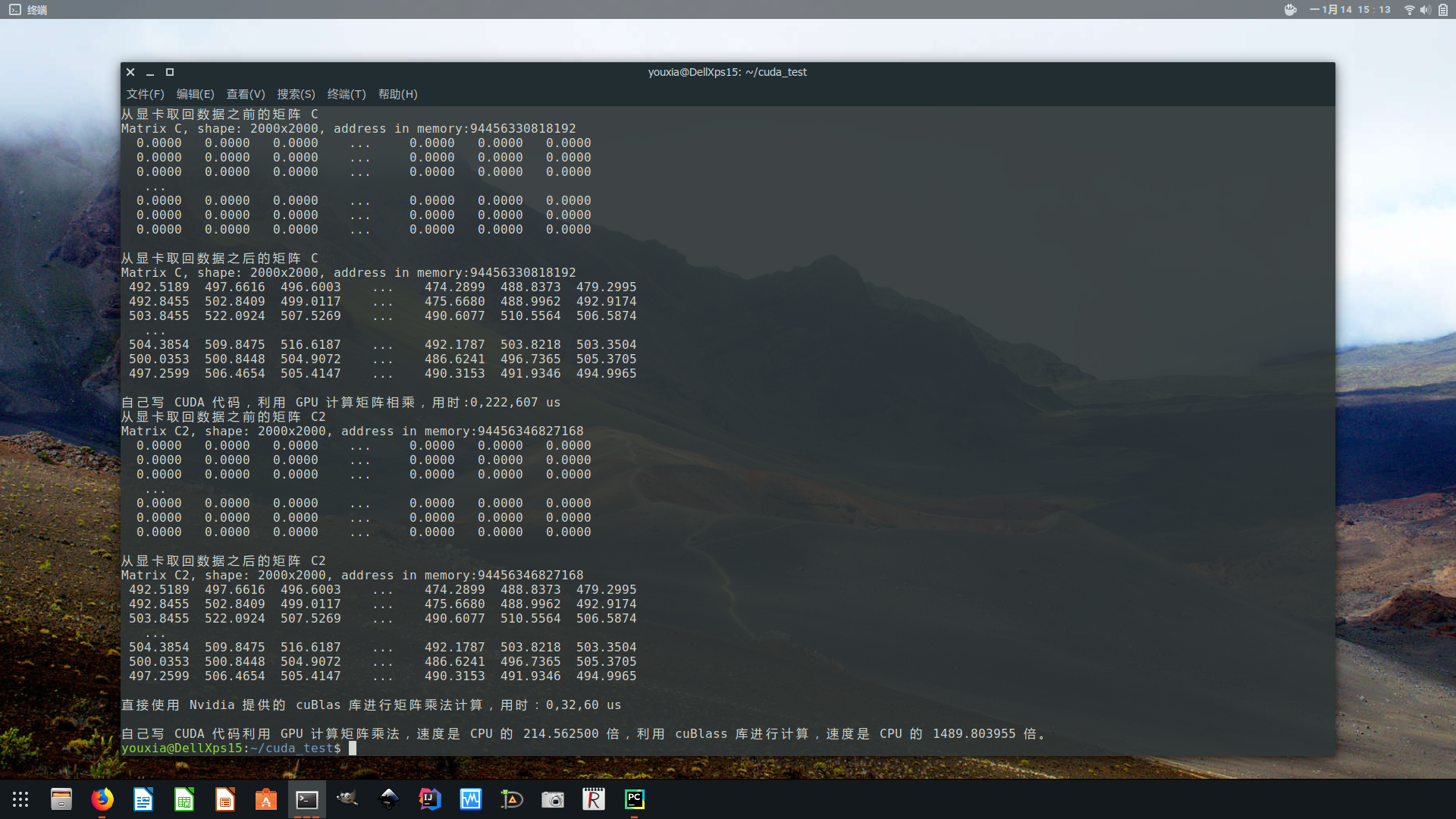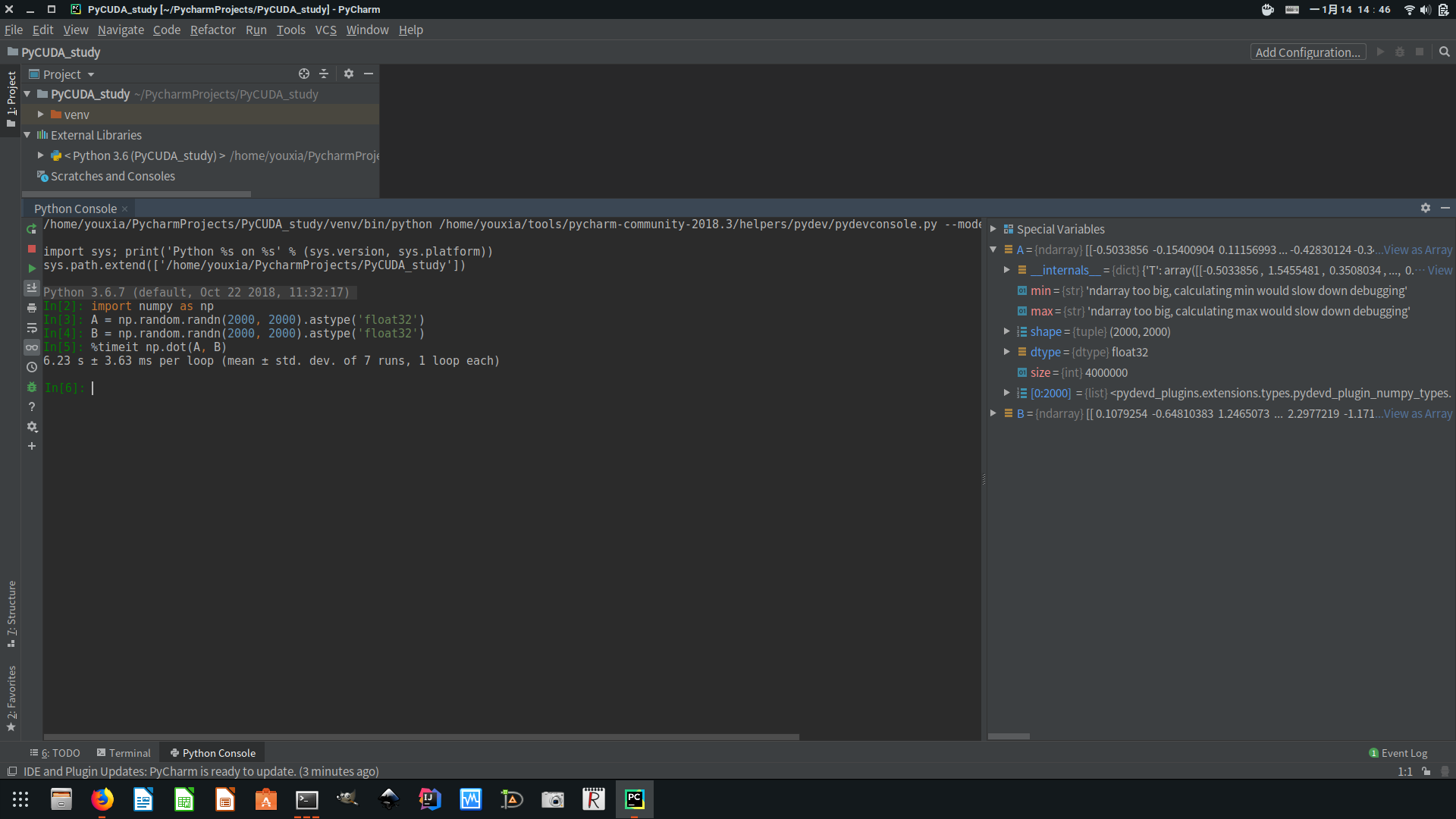import numpy as np
A = np.random.randn(2000, 2000).astype('float32')
B = np.random.randn(2000, 2000).astype('float32')
%timeit np.dot(A, B)

开发环境的安装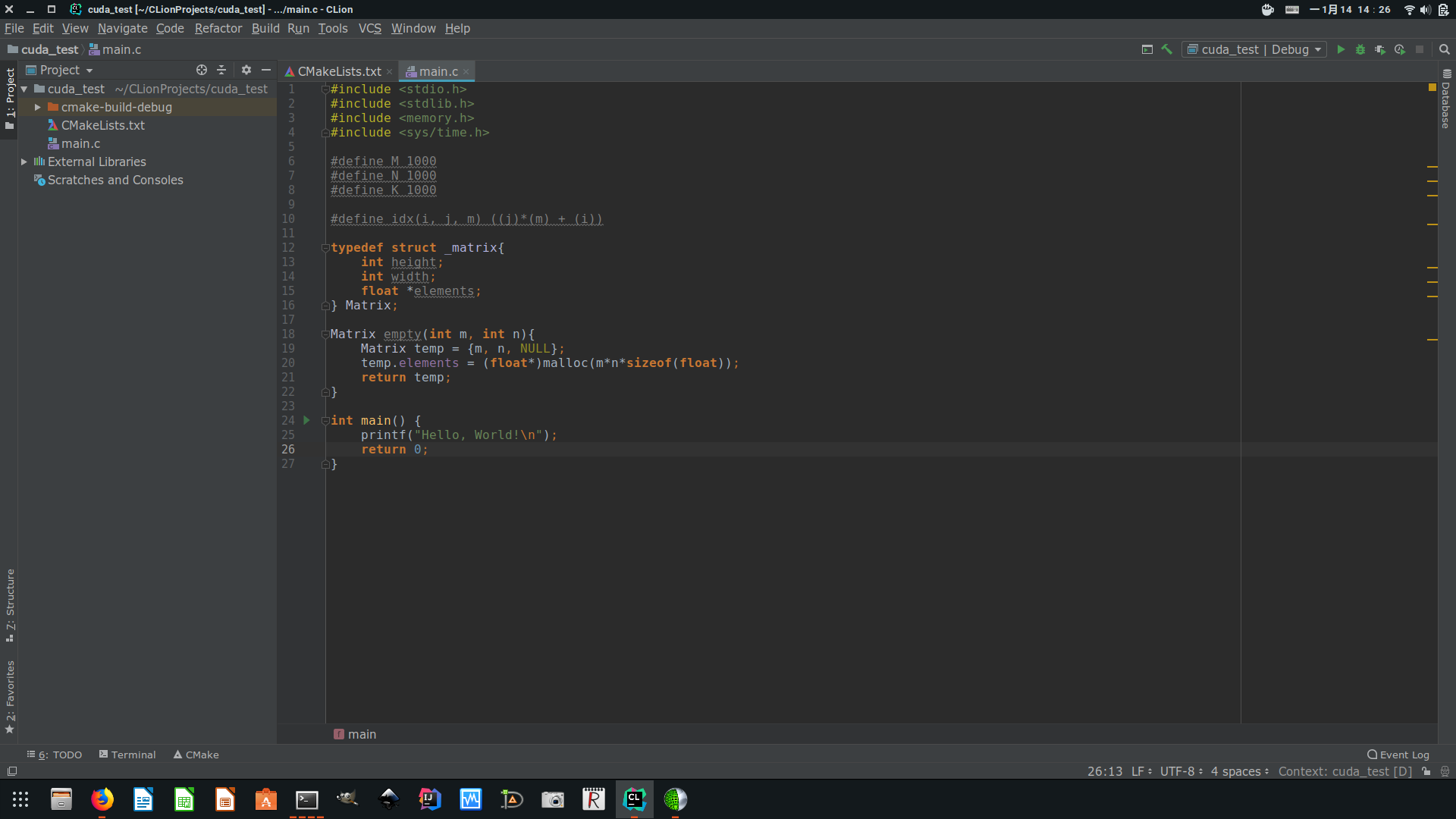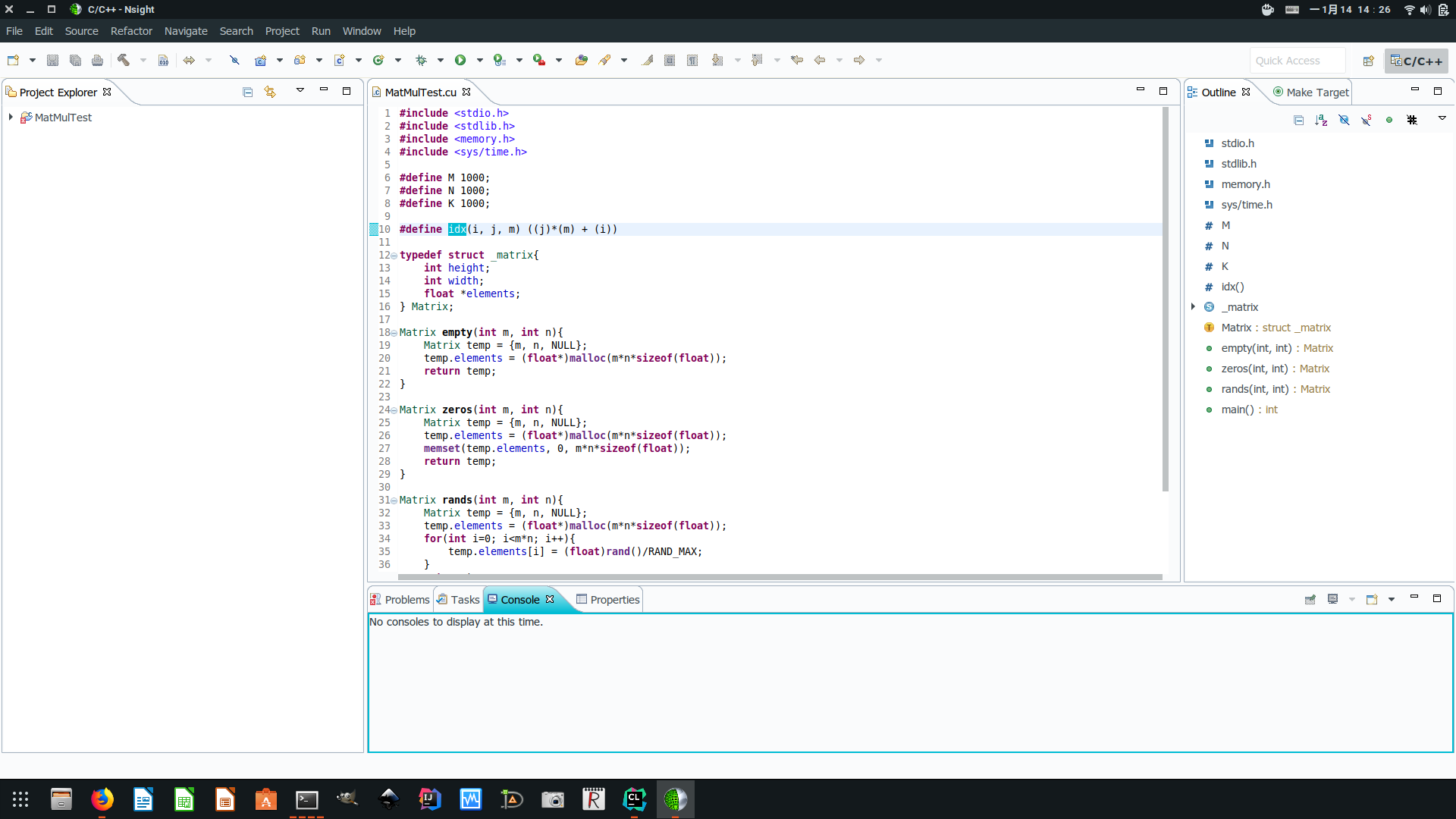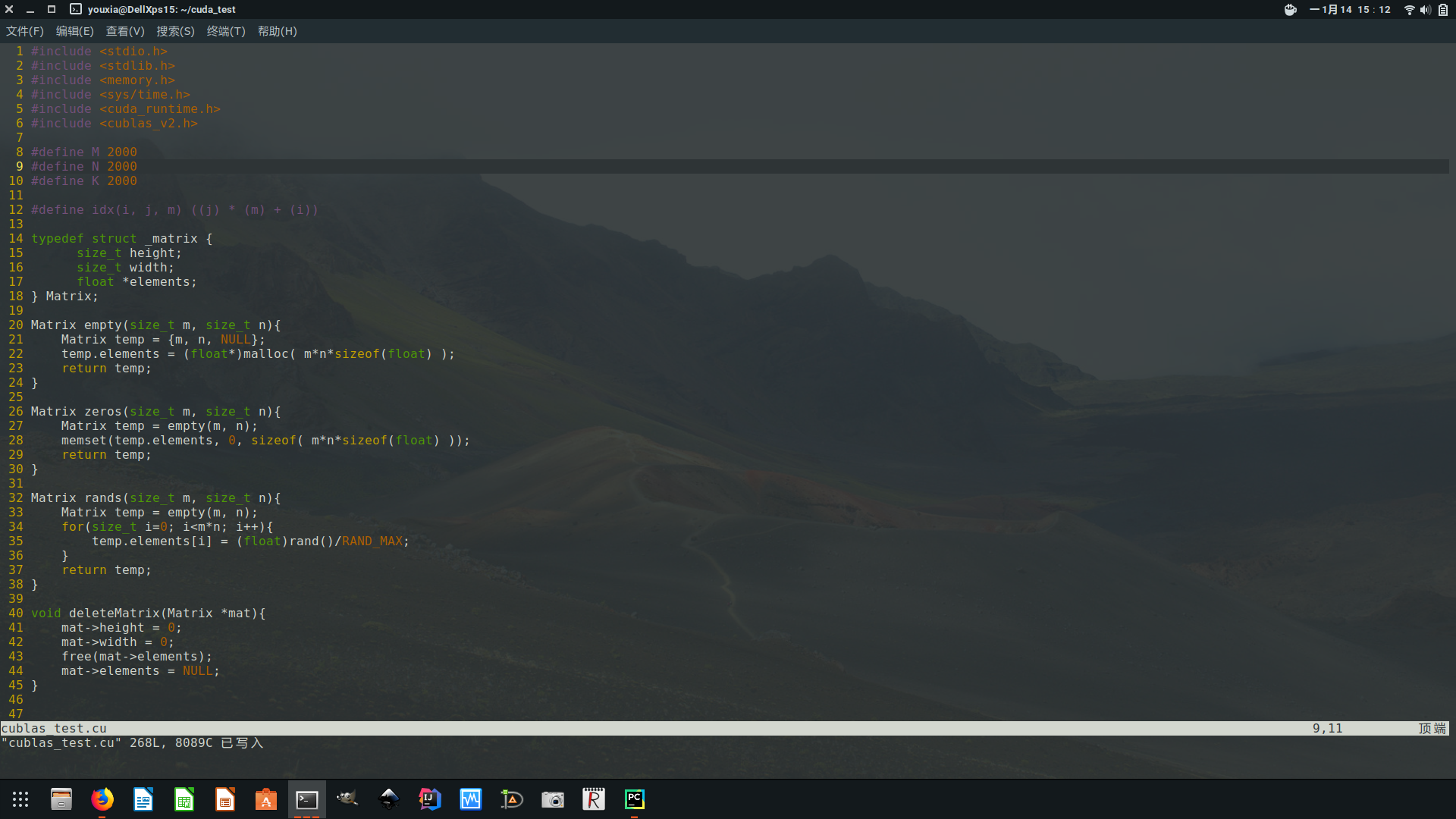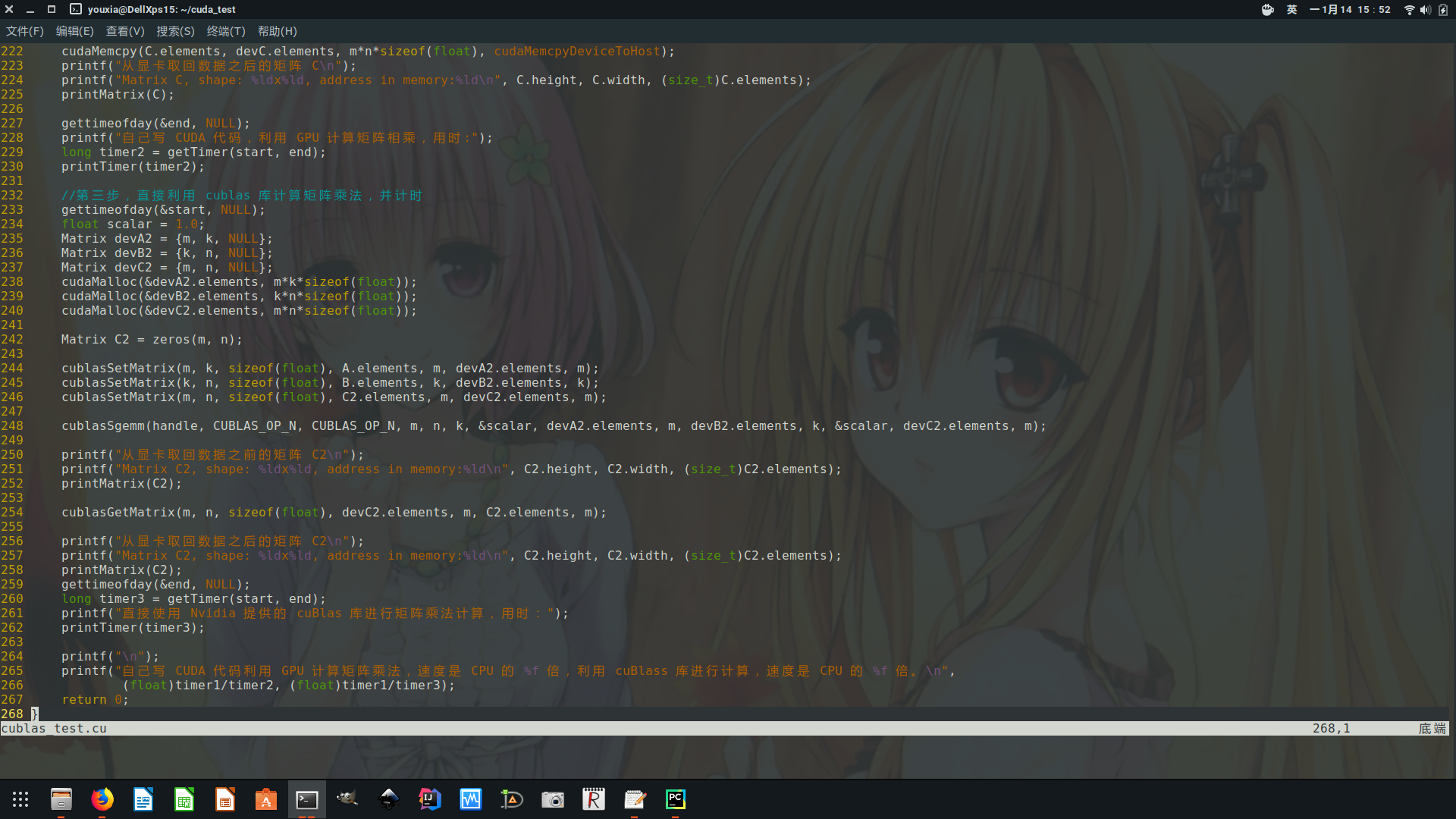代码解释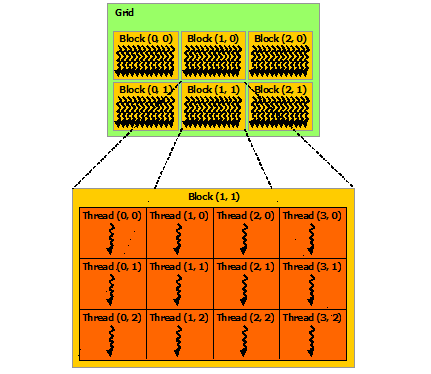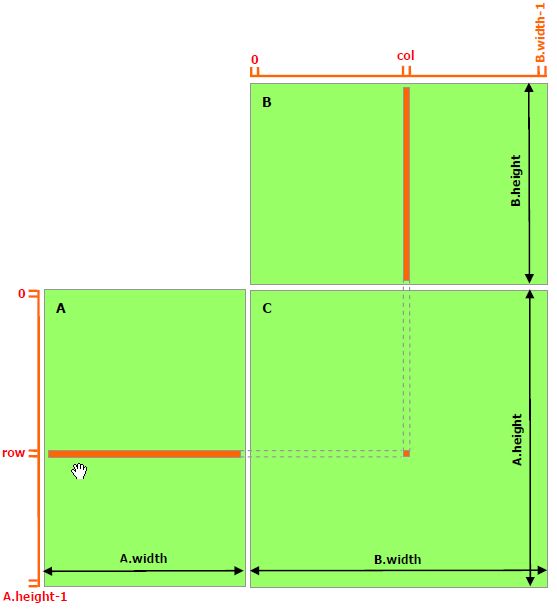CUDA 中每个 block 中的 thread 数是有上限的，在我的电脑上，该上限是 1024。所以我定义blockSize = 32，然后 block 的形状就是(blockSize, blockSize)，也就是 block 的大小为 32×32。然后再把 grid 的形状定义为((m + blockSize -1)/blockSize, (n + blockSize -1)/blockSize)，这样，这个 grid 中的总线程数就是 M×N 了，而且线程排列的形状和结果矩阵的形状完全一样，所以每个线程计算结果矩阵中的一个元素，极其方便。

    Matrix A = rands(M, K);
printf("Matrix A, shape: %ldx%ld, address in memory:%ld\n", A.height, A.width, (size_t)A.elements);
printMatrix(A);

Matrix B = rands(K, N);
printf("Matrix B, shape: %ldx%ld, address in memory:%ld\n", B.height, B.width, (size_t)B.elements);
printMatrix(B);

Matrix C = matMul(A, B);
printf("Matrix C, shape: %ldx%ld, address in memory:%ld\n", C.height, C.width, (size_t)C.elements);
printMatrix(C);

    size_t m = A.height;
size_t n = B.width;
size_t k = B.height;
Matrix devA = {m, k, NULL};
Matrix devB = {k, n, NULL};
Matrix devC = {m, n, NULL};

    cudaMalloc(&devA.elements, m*k*sizeof(float));
cudaMemcpy(devA.elements, A.elements, m*k*sizeof(float), cudaMemcpyHostToDevice);
cudaMalloc(&devB.elements, k*n*sizeof(float));
cudaMemcpy(devB.elements, B.elements, k*n*sizeof(float), cudaMemcpyHostToDevice);
cudaMalloc(&devC.elements, m*n*sizeof(float));
cudaMemset(devC.elements, 0, m*n*sizeof(float));

    size_t blockSize = 32;
size_t gridWidth = (n + blockSize -1)/blockSize;
size_t gridHeight = (m + blockSize -1)/blockSize;
cudaMatMul<<<dim3(gridHeight, gridWidth), dim3(blockSize, blockSize)>>>(devA, devB, devC);

Kernel 函数cudaMatMul()是怎么定义的呢？如下：

__global__ void cudaMatMul(Matrix devA, Matrix devB, Matrix devC){
size_t m = devA.height;
size_t k = devB.height;
size_t n = devB.width;

size_t j = blockIdx.y * blockDim.y + threadIdx.y;
size_t i = blockIdx.x * blockDim.x + threadIdx.x;

if(i<m && j<n){
float sum = 0.0;
for(size_t p=0; p<k; p++){
sum += devA.elements[idx(i, p, m)] * devB.elements[idx(p, j, k)];
}
devC.elements[idx(i, j, m)] = sum;
}
}

    deleteMatrix(&C);
C = zeros(m, n);
printf("从显卡取回数据之前的矩阵 C\n");
printf("Matrix C, shape: %ldx%ld, address in memory:%ld\n", C.height, C.width, (size_t)C.elements);
printMatrix(C);
cudaMemcpy(C.elements, devC.elements, m*n*sizeof(float), cudaMemcpyDeviceToHost);
printf("从显卡取回数据之后的矩阵 C\n");
printf("Matrix C, shape: %ldx%ld, address in memory:%ld\n", C.height, C.width, (size_t)C.elements);
printMatrix(C);

cuBLAS 使用<cublas_v2.h>头文件，编译时使用-lcublas连接相应的库。为什么头文件中有个v2呢？这一因为这个新版本是线程安全的，所以每个 cuBLAS 函数都需要一个handle作为参数，这个handle可以使用cublasCreate()函数创建，不同的线程可以使用不同的handle。我把创建handle()的工作也放到 CUDA 初始化的代码里面了，在initCUBLAS()函数中。